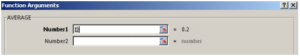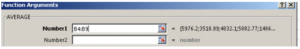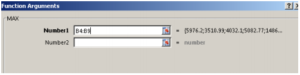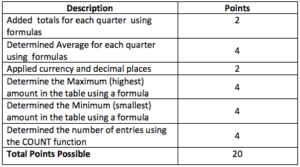## Public Expenses by Quarter Assignment

### The purpose of this assignment is to:

1. Format Cell Content
2. Use formulas for Min, Max, Ave, Count

### Items to Review from previous lessons

• AutoSum Key
• Auto Fill key
• Currency and Decimal Points

### New Terms:

• AVE – Average a row or column of numbers (Step , a-g) (Pages 1-2)
• MAX – Maximum – the largest number in a row or column (Step 5, a-e) (Page 2)
• MIN – Minimum – the smallest number in a row or column (Step 6a) (Page 2)
• Count – Count the number of entries in a row or column (Step 7) (Pages 2-3)

### Narrative:

Understanding how simple formulas work is a building step in working with spreadsheets. These four formulas (Ave, Max, Min, Count) can quickly calculate information. (THIS ASSIGNMENT IS WORTH 20 POINTS. THE RUBRIC IS ON PAGE 3)

### Assignment:

2. Put your name in cell A24
3. Using the AutoSum Key, determine the totals for each Quarter.
4. AVE. What is the Average (AVE) quarterly expense? Follow steps a-d below.
• Click in cell B12
• Click on FORMULA tab, the click More Functions in the Function Library Group and click on Statistical. Find AVERAGE.
• An argument box appears as pictured below.• In the space called Number 1, the computer is looking for what you want averaged.
• Select in your worksheet B4:B9. This selection will show after Number 1.• Enter and the total should be: 4547.22833.
• Currency and Decimals. Select the answer. Change it to two decimal places by clicking on the HOME tab, click on the number launcher button in the number group. Then click on the selection Number that follows the word GENERAL. Change to 2 decimal places. Your answer should now be 4547.23.

5. MAX. What is the Maximum (the largest) amount in a list of numbers?

• Click in Cell B13
• Click on FORMULA tab. Click More Functions in the Function Library Group and click on Statistical. Find MAX for maximum. (They functions are all in alphabetic order so scroll down until you find it.)
• An argument box appears as pictured below, and looks similar to the one for Average.• Select cells B4:B9. ENTER. (Do not put any information in “Number 2” box.) e. The answer should be 7195.06.

6. MIN. What is the Minimum (lowest amount) in the list of numbers?

• Click on FORMULA tab. Click More Functions in the Function Library Group and click on Statistical. Find MAX for maximum. (They functions are all in alphabetic order so scroll down until you find it.)
• An argument box appears as pictured above in 3d but the Function Arguments are for MIN, and looks similar to the one for Average.
• Select cells B4:B9. ENTER. (Do not put any information in “Number 2” box.)
• The answer should be 1486.25.

7. Count. How can I Count the number of items in a list?

• Click on FORMULA tab and look to the left for another AutoSum Key. This is the same function, just in a different space.
• Click on the arrow beside the AutoSum Key to see some options. One of those options is Count.
• When you click on Count, you do not get the argument box as you have with the other functions. However, the formula is similar to MAX and MIN.
• Instead of using the Argument Box, type an equal sign followed by the word COUNT (in all caps) followed by an open parenthesis
• Select cells B4:B9 with your mouse so that these cell references become part of the formula. (Notice the formula in the formula box.
• Close the parenthesis and press the Enter key.